Register for Maths, Science, English, Reasoning Olympiad Exams Register here | Book Free Demo for Live Olympiad Classes here | Check Olympiad Exam Dates here | Buy Practice Papers for IMO, IOM, HEO, IOEL etc here | Login here to participate in all India free mock test on every Saturday

# Measurement-Time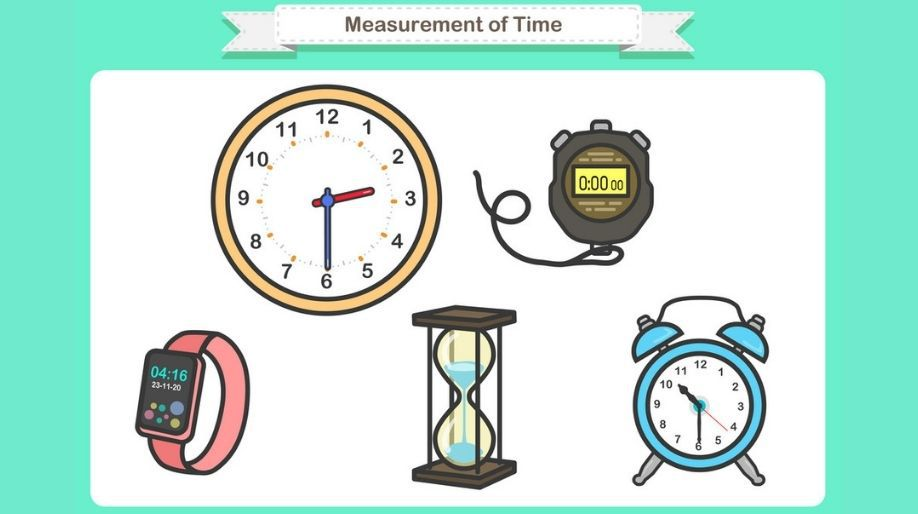Measurement-Time

Units of Time: Here are the units that we use in our daily life to check the time.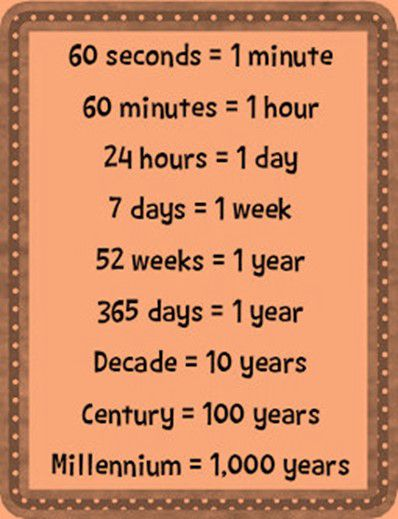Time Interval: Time interval is defined as period between two points of time.

For example: The time interval between 12 o’ clock and 1 o’clock is 1 hour.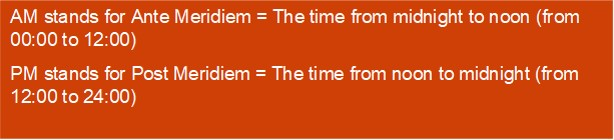Conversion of Minutes into Hours: Follow the given steps

• Divide the number of minutes by 60
• Quotient defines the number of hours.
• The remainder is number of minutes.

For example: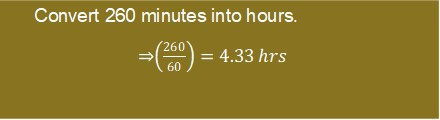Conversion of Seconds into Minutes:

• Divide the number of seconds by 60.
• The quotient defines the number of minutes.
• The remainder defines the number of seconds greater than full minutes.

For example: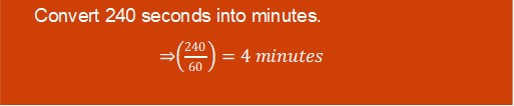Addition and Subtraction of Time: Always add or subtract the hours, minutes and seconds separately.

For example: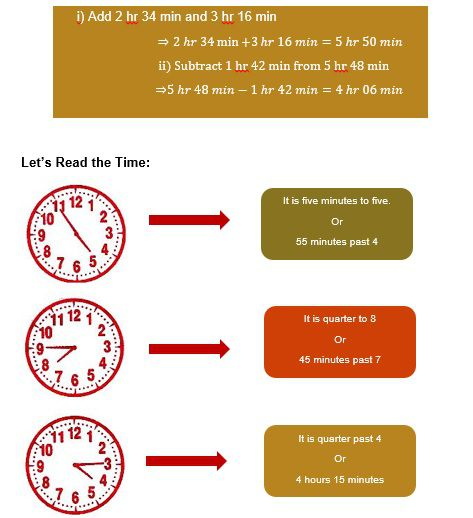Exercise

• Convert following into hours:
1. 230 minutes
2. 346 minutes
• 198 minutes

• Convert following into minutes:
1. 240 seconds
2. 360 seconds
• 196 seconds

1. 4 hrs 16 min and 6 hrs 20 min
2. 6 hrs 09 min and 7 hrs 19 min
• 2 hrs 18 min and 3 hrs 22 min

• Subtract the following:
1. 4 hrs 34 min from 6 hrs 44 min
2. 1 hrs 25 min from 4 hrs 13 min
• 3 hrs 44 min from 8 hrs 60 min
• Tell the time in the following watch: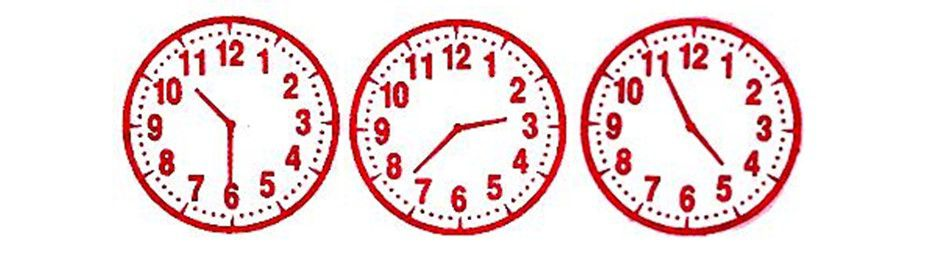Recap

• 1 minute = 60 seconds, 1 hour = 60 minutes and 1 day = 24 hours
• 1 day = 24 hours, 1 week = 7 days and 1 year = 12 months/ 365 days
• Calendar is a chart to represent the months, weeks and days.
• Leap Year has 366 days.

## Quiz for Measurement-Time

 Q.1 What is the time in the analog watch?a) 11:05 b) 11:15 c) 11:30 d) 11:45
 Q.2 What is the time in the analog watch?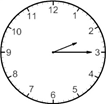a) 2:10 b) 2:00 c) 2:25 d) 2:15
 Q.3 What is the time in the analog watch?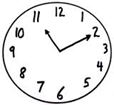a) 11:10 b) 11:05 c) 11:15 d) 10:10
 Q.4 Which clock shows time 10:25? a)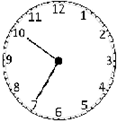b)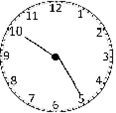c)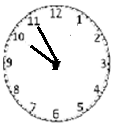d)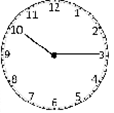Q.5 Which clock shows time 6:35? a)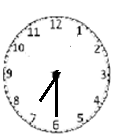b)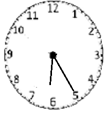c)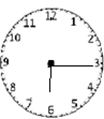d)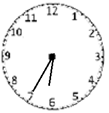Q.6 Which clock shows time 12:25? a)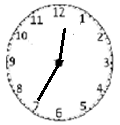b)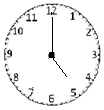c)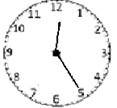d)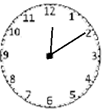Q.7 Fill in the blanks(determine the total elapsed time):- 4:30 + ____?_____ = 5:45 a) 2 hours 15 minutes b) 1 hour 45 minutes c) 1 hour 15 minutes d) 1 hour 30 minutes
 Q.8 Fill in the blanks(determine the total elapsed time):- 3:10+ ____?_____= 4:55 a) 1 hour 55 minutes b) 1 hour 45 minutes c) 1 hour 10 minutes d) 2 hours 45 minutes
 Q.9 Fill in the blanks(determine the total elapsed time):- 12:10+ ____?____= 12:55 a) 1 hour 15 minutes b) 1 hour 45 minutes c) 45 minutes d) 35 minutes
 Q.10 Fill in the blanks(determine the total elapsed time):- 1:12+_____?____= 4:29 a) 2 hours 17 minutes b) 3 hours 17 minutes c) 2 hours 27 minutes d) 3 hours 27 minutes